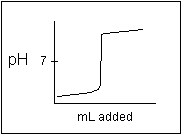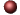Aqueous Equilibrium - Buffers & TitrationsExamples ofMultiple Choice Questions

1.
Which of the following combinations cannot produce a buffer solution?
(a) HNO2 and NaNO2
(b) HCN and NaCN
(c) HClO4 and NaClO4
(d) NH3 and (NH4)2SO4
(e) NH3 and NH4Br

2.
What is the pH of a solution composed of 0.20 M NH3 and 0.15 M NH4Cl?
(a) 2.15
(b) 4.62
(c) 8.26
(d) 9.38
(e) 8.89

3.
Calculate the ratio [CH3COOH]/[NaCH3COO] that gives a solution with pH = 5.00?
(a) 0.28
(b) 0.36
(c) 0.44
(d) 0.56
(e) 0.63

4.
Consider a solution which is 0.10 M in CH3COOH and 0.20 M in NaCH3COO. Which of the following statements is true?

(a) If a small amount of NaOH is added, the pH decreases very slightly.
(b) If NaOH is added, the OH- ions react with the CH3COO- ions.
(c) If a small amount of HCl is added, the pH decreases very slightly.
(d) If HCl is added, the H+ ions react with CH3COOH ions.
(e) If more CH3COOH is added, the pH increases.

5.
A buffer was prepared by mixing 1.00 mole of ammonia and 1.00 mole of ammonium chloride to form an aqueous solution with a total volume of 1.00 liter. To 500 mL of this solution was added 30.0 mL of 1.00 M NaOH. What is the pH of this solution?
(a) 8.96
(b) 9.83
(c) 9.31
(d) 9.11
(e) 9.57

6.
How many grams of NaF would have to be added to 2.00 L of 0.100 M HF to yield a solution with a pH = 4.00?
(a) 300 g
(b) 36 g
(c) 0.84 g
(d) 6.9 g
(e) 60. g

7.
Calculate the pH that results when the following solutions are mixed.
(1) 35 mL of 0.20 M formic acid
(2) 55 mL of 0.10 M sodium formate
(3) 110 mL of water

(a) 3.64
(b) 3.11
(c) 4.58
(d) 3.39
(e) 4.20

8.
Consider an indicator that ionized as shown below for which its Ka = 1.0 x 10-4
 HIn + H2OH3O+ + In- yellow red
Which of the responses contains all the true statements and no others?

(1) The predominant color in its acid range is yellow.
(2) In the middle of the pH range of its color change a solution containing the indicator will probably be orange.
(3) At pH = 7.00, a solution containing this indicator (and no other colored species) will be red. (Hint: Write the equilibrium constant expression for the indicator.)
(4) At pH = 7.00, most of the indicator is in the un-ionized form.
(5) The pH at which the indicator changes color is pH = 4.

(a) 1, 3, 5
(b) 2, 4
(c) 3, 4, 5
(d) 1, 2, 3, 5
(e) another combination

9.
Calculate the pH of the solution resulting from the addition of 20.0 mL of 0.100 M NaOH to 30.0 mL of 0.100 M HNO3.
(a) 1.35
(b) 1.70
(c) 1.95
(d) 2.52
(e) 2.80

10.
Which indicator (identified by a letter) could be used to titrate aqueous NH3 with HCl solution?
 Indicator Acid Range Color Color-Change pH Basic Range Color (a) pink 1.2 - 2.8 yellow (b) blue 3.4 - 4.6 yellow (c) yellow 6.5 - 7.8 purple (d) colorless 8.3 - 9.9 red (e) none of these

11.
Consider the titrations of the pairs of aqueous acids and bases listed on the left. For which pair is the pH at the equivalence point stated incorrectly?
 Acid-Base Pair pH at Equivalence Point (a) HCl + NH3 less than 7 (b) HNO3 + Ca(OH)2 equal to 7 (c) HClO4 + NaOH equal to 7 (d) HClO + NaOH less than 7 (e) CH3COOH + KOH greater than 7

12.
What is the pH at the equivalence point in the titration of 100.0 mL of 0.20 M ammonia with 0.10 M hydrochloric acid?
(a) 4.6
(b) 5.2
(c) 7.0
(d) 5.5
(e) 4.9

13.
Calculate the pH of a solution prepared by mixing 300 mL of 0.10 M HF and 200 mL of 0.10 M KOH.
(a) 2.82
(b) 2.96
(c) 3.32
(d) 3.44
(e) 3.53

14.
What is the approximate pH of a solution prepared by mixing equal volumes of 0.05 M methylamine and 0.20 M hydrochloric acid?
(a) 2.57
(b) 1.12
(c) 1.63
(d) 10.5
(e) 9.8

15.
Which of the following salts give acidic aqueous solutions?
 (1) KNO3 (2) KCH3COO (3) NH4NO3 (4) RbI (5) (NH4)2SO4 (6) BaCl2 (7) NaCN (8) KNO2
(a) 2, 7, 8
(b) 3, 5
(c) 2, 4, 6
(d) 1, 4, 7, 8
(e) 1, 4, 6

16.
The following titration curve is the kind of curve expected for the titration of a ____ acid with a ____ base.(a) strong, strong
(b) weak, strong
(c) strong, weak
(d) weak, weak
(e) none of these

17.
Consider the titration of 30.0 mL of 0.20 M nitrous acid by adding 0.0500 M aqueous ammonia to it. The pH at the equivalence point is _____. (Note: This is the titration of a weak acid with a weak base.)
(a) greater than 7
(b) equal to 7
(c) less than 7
(d) cannot be determined without more data (not including Ka and Kb)
(e) is impossible to predict

1. (c) 2. (d) 3. (d) 4. (c) 5. (c) 6. (e) 7. (a) 8. (d) 9. (b) 10. (b) 11. (d) 12. (b) 13. (d) 14. (b) 15. (b) 16. (a) 17. (c)Click here to return to the top.Choose your next chapter:
Fundamentals of Chemistry | Chemical Formulas & Composition Stoichiometry | Chemical Equations & Rxn Stoichiometry | Types of Chemical Reactions |
| Atomic Structure | Chemical Periodicity | Chemical Bonding | Molecular Structure/Covalent Bonding Theories| Molecular Orbital Theory |
| Acids/Bases/Salts - Theory & Rxns | Acids/Bases/Salts - Calculations (including balancing redox rxns) | Gases | Solids & Liquids | Solutions |
| Thermodynamics | Kinetics | Equilibrium | Aqueous Equilibrium - Acids/Bases/Salts | Aqueous Equilibrium - Buffers & Titrations |
| Aqueous Equilibrium - Slightly Soluble Salts | Electrochemistry | Metallurgy | Metal Properties & Rxns | Nonmetals & Metalloids |
| Coordination Compounds | Nuclear Chemistry | Organic Chem - Formulas/Names/Properties | Organic Chem - Shapes/Rxns/Biopolymers |To report any corrections, please e-mail Dr. Wendy Keeney-Kennicutt.